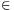Mathematical and Physical Journal
for High Schools
Issued by the MATFUND Foundation
 Already signed up? New to KöMaL?

#Problem A. 528. (February 2011)

A. 528. Let a, b and n be positive integers and let C be a finite set of integers. Suppose that every positive integer can be represented in the form axn+byn+c with some positive integers x, y and cC. Find the possible values of n.

(Proposed by Péter Kutas, Budapest)

(5 pont)

Deadline expired on March 10, 2011.

### Statistics:

 7 students sent a solution. 5 points: Backhausz Tibor, Mester Márton, Nagy 235 János, Nagy 648 Donát, Strenner Péter. 3 points: 1 student. 2 points: 1 student.

Problems in Mathematics of KöMaL, February 2011